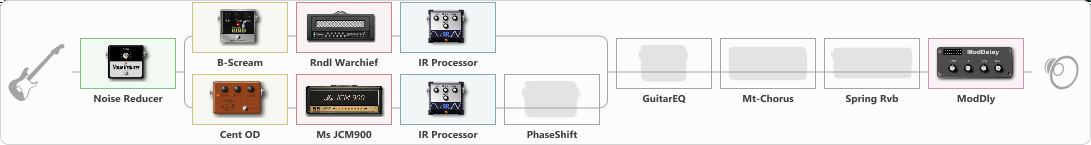# Dual

Discussion in 'ToneLib-GFX presets' started by carlinos3, May 18, 2023.

1. Dual

Effects chain:Effect: "Noise Reducer" (Dynamics / Filter), active - "yes"
{
"Sens" = 69
"Mode" = Hard
}

Effect: "Splitter" (Dynamics / Filter)
{
"A-Bypass" = Off
"A-Pan" = 65
"A-Level" = 66
"B-Bypass" = Off
"B-Pan" = -65
"B-Level" = 66
"Width" = 0

'A' branch:
{

Effect: "B-Scream" (Overdrive / Distortion), active - "yes"
{
"Drive" = 67
"Tone" = 55
"Level" = 60
}

Effect: "Rndl Warchief" (Amp simulators), active - "yes"
{
"Gain" = 100
"Bass" = 50
"Middle" = 50
"Treble" = 50
"Presence" = 50
"Master" = 100
"Level (dB)" = 0
}

Effect: "IR Processor" (Cabinets), active - "yes"
{
"IR" = dic-1_dc
"Low Cut (Hz)" = 0
"Hi Cut (kHz)" = 20.0
"Mix" = 100
"Level (dB)" = 0
}
}
'B' branch:
{

Effect: "Cent OD" (Overdrive / Distortion), active - "yes"
{
"Drive" = 38
"Tone" = 76
"Level" = 38
}

Effect: "Ms JCM900" (Amp simulators), active - "yes"
{
"Gain" = 100
"Bass" = 50
"Middle" = 50
"Treble" = 50
"Presence" = 50
"Master" = 100
"Level (dB)" = 0
}

Effect: "IR Processor" (Cabinets), active - "yes"
{
"IR" = barc_dc
"Low Cut (Hz)" = 0
"Hi Cut (kHz)" = 20.0
"Mix" = 100
"Level (dB)" = 0
}

Effect: "PhaseShift" (Modulation / Sfx), active - "no"
{
"Shift" = 55
"Mix" = 100
}
}
}

Effect: "GuitarEQ" (Dynamics / Filter), active - "no"
{
"160 Hz" = 0
"400 Hz" = 0
"800 Hz" = 0
"1.6 kHz" = 0
"3.2 kHz" = 0
"6.4 kHz" = 0
"12 kHz" = 0
"Level (dB)" = 2
}

Effect: "Mt-Chorus" (Modulation / Sfx), active - "no"
{
"Speed" = 1.8
"Depth" = 23
"Time" = 1.4
"Mix" = 28
}

Effect: "Spring Rvb" (Reverberation), active - "no"
{
"Time" = 7.0
"PreDelay" = 0
"LoDamp" = 16
"HiDamp" = 26
"Mix" = 42
}

Effect: "ModDly" (Delay), active - "yes"
{
"Time" = 508
"Feedback" = 1
"Tone" = 66
"Speed" = 1.7
"Mix" = 48
}

Note: You will need to download and install the ToneLib-GFX software to use the preset.

#### Attached Files:

• ###### Dual.tlgfx
File size:
129.7 KB
Views:
361
Nikolay5050NIKOLAY likes this.